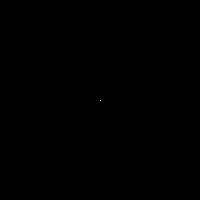# Create and save animated GIF with Python, Pillow

Posted: 2019-05-14 / Tags: Python, Pillow, Image Processing

Using the Python image processing library Pillow (PIL), you can create and save animated GIFs.

• Save as GIF with `Image.save()`
• Sample code to generate animated GIF
• Parameters of `Image.save()`
• `append_images`
• `optimize`
• `loop`
• `duration`

## Save as GIF with `Image.save()`

You can create an animated GIF from multiple images and save it using `Image.save()`.

``````im.save('out.gif', save_all=True, append_images=[im1, im2, ...])
``````

The image list `[im1, im2, ...]` is added to the image `im` of the first frame, and an animated GIF file `out.gif` is generated and saved.

See the official document below for details.

The sample code and the explanation of the parameters are shown below.

## Sample code to generate animated GIF

Here is sample code for drawing circles that grows gradually with Pillow's `ImageDraw` and saving it as a GIF file.

``````from PIL import Image, ImageDraw

images = []

width = 200
center = width // 2
color_1 = (0, 0, 0)
color_2 = (255, 255, 255)
step = 8

for i in range(0, max_radius, step):
im = Image.new('RGB', (width, width), color_1)
draw = ImageDraw.Draw(im)
draw.ellipse((center - i, center - i, center + i, center + i), fill=color_2)
images.append(im)

for i in range(0, max_radius, step):
im = Image.new('RGB', (width, width), color_2)
draw = ImageDraw.Draw(im)
draw.ellipse((center - i, center - i, center + i, center + i), fill=color_1)
images.append(im)

images.save('data/dst/pillow_imagedraw.gif',
save_all=True, append_images=images[1:], optimize=False, duration=40, loop=0)
``````

The following animated GIF file is generated.Refer to the following article about how to draw figures such as circles with Pillow.

## Parameters of `Image.save()`

Basically, if you have a list of images you can create an animated GIF with the following code:

``````images.save('data/dst/pillow_imagedraw.gif',
save_all=True, append_images=images[1:], optimize=False, duration=40, loop=0)
``````

If you want to create a GIF animation from multiple existing images instead of drawing graphics and creating images as in the example above, you can process the same if you load the images and store them in a list.

### `append_images`

Call the `save()` method from the first frame image, passing the list of remaining images to `append_images`.

Note that if you use `append_images=images`, the first frame will be repeated twice. In the above example, the second and subsequent images are selected by slicing.

### `optimize`

I have not read the source code properly, so I do not know what processing is done, but if the default (`optimize=True`), similar images may be omitted.

If the output file is wrong, trying `optimize=False` may solve it.

### `loop`

Set the number of loops to `loop`. If you set `loop=0`, it becomes an infinite loop.

Note that the animation ends in one time by default.

### `duration`

Set the display time of each frame to `duration` in milliseconds. If the value is too small, it will be ignored.